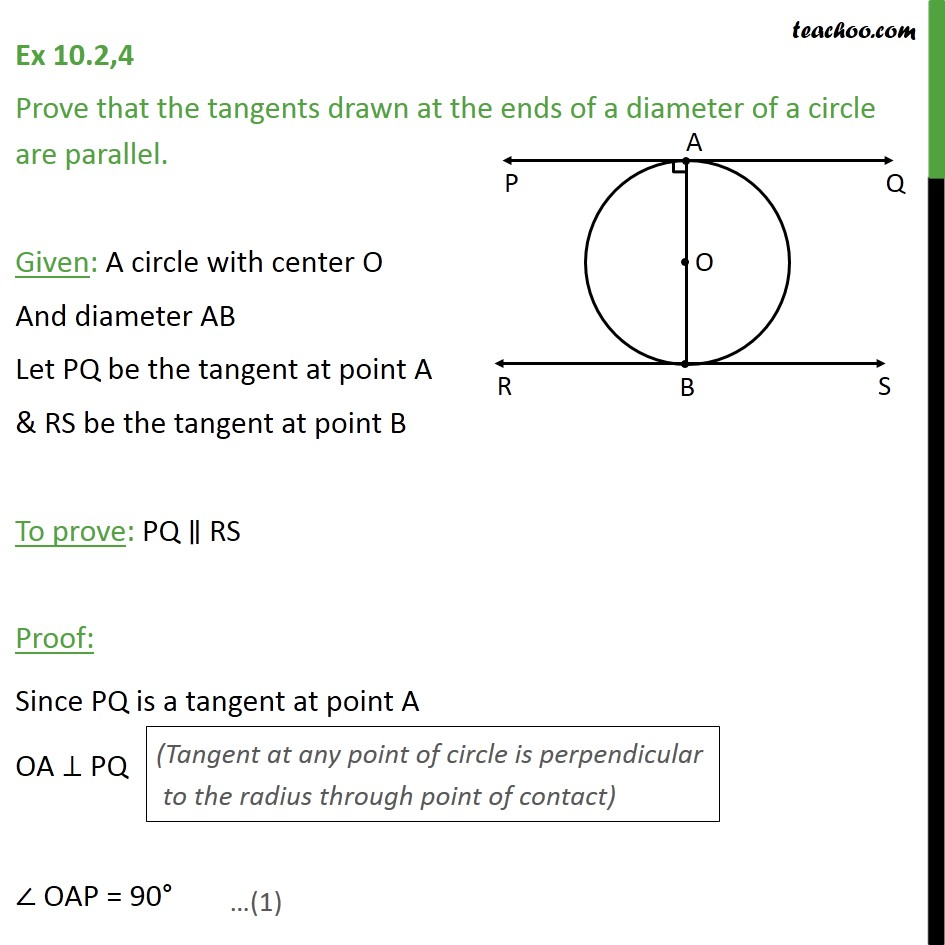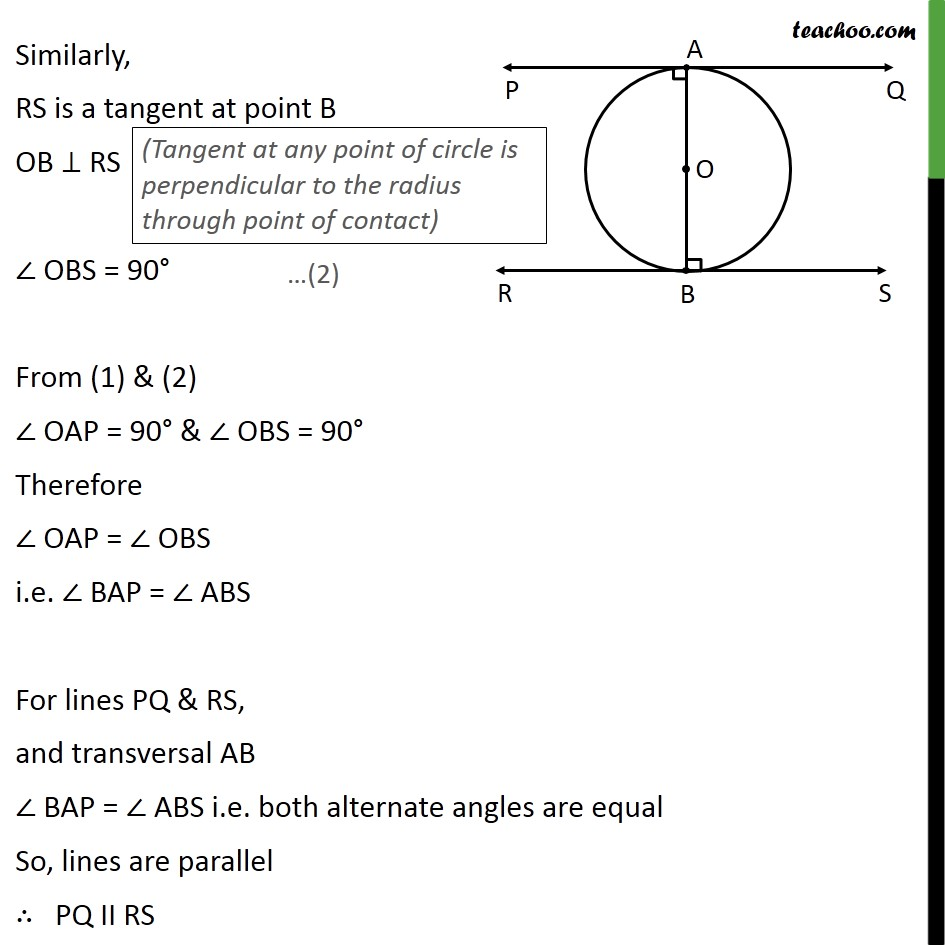Theorem 10.1: Tangent perpendicular to radius (proof type)Learn in your speed, with individual attention - Teachoo Maths 1-on-1 Class

### Transcript

Ex 10.2,4 Prove that the tangents drawn at the ends of a diameter of a circle are parallel. Given: A circle with center O And diameter AB Let PQ be the tangent at point A & RS be the tangent at point B To prove: PQ ∥ RS Proof: Since PQ is a tangent at point A OA ⊥ PQ ∠ OAP = 90° Similarly, RS is a tangent at point B OB ⊥ RS ∠ OBS = 90° From (1) & (2) ∠ OAP = 90° & ∠ OBS = 90° Therefore ∠ OAP = ∠ OBS i.e. ∠ BAP = ∠ ABS For lines PQ & RS, and transversal AB ∠ BAP = ∠ ABS i.e. both alternate angles are equal So, lines are parallel ∴ PQ II RS